# [OpenGL coordinate systems]

Good morning,

I tried to rotate an object using quaternion.
So for example when I set the values of the quaternion as follows:
w=0.7
x=0.7
y=0
z=0
the object rotates around the x axis of a certain coordinate system which I think, is the world’s coordinate system.
[The Euler angles that corresponds to this quaternion are rotation around x axis =90, rotation around y axis=0 and rotation around z=0].
When I change the orientation of the cooridnate system’s axes and apply the rotation, the object rotates around the old coordinate system. I mean, all I want to do is change the orientation of the x axis for example, using glrotated before drawing the frame
then apply the rotation using the values of a quaternion for example: [w=0.7,x=0.7,y=0,z=0] and make the object rotate around the new x axis.
Is that even possible ? Can you please provide me with some tips on how to do it ?

Order of operations matters. When reading a product left to right, each operation is interpreted in the coordinate system established by previous operations (those to its left). So if X is a rotation about the X axis and T is the current transformation, T=X*T rotates about the global X axis, T=T*X rotates about the local X axis established by T.

I’m sorry, I didn’t really understand since I’m new to OpenGL. Can you please re-explain ?
But here is exactly what I have done:
In my paintGL function:
I defined a quaternion, normalized it, determined the rotation matrix then used this function glMultMatrixf(rot); where rot is the rotation matrix, to rotate the object.
Then I drew the box ( the object I want to rotate), next Idrew the frame or the coordinate system.

So this is my initial object orientation :When I change the values of the quaternion (w=0.7,x=0,y=0,z=0.7) the object rotates around z axis [since this quaternion corresponds to a 90°rotation around z axis] as shown below: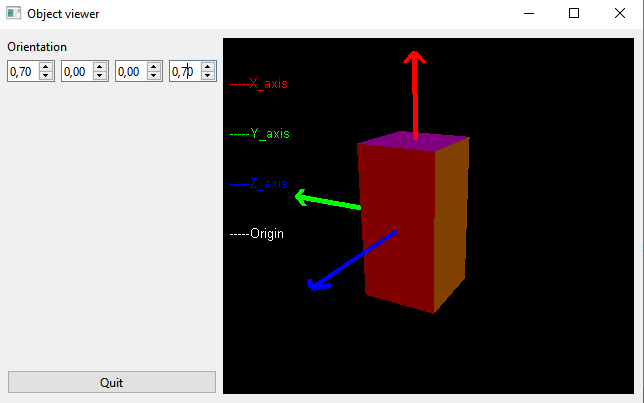Now if I put glRotatef(-90.0f,0.0,1.0,0.0); before drawing the frame,to change x and z orientations and run my application, change the values of the quaternion, the object doesn’t rotate around the new z axis ( which orientation is changed) as shown below: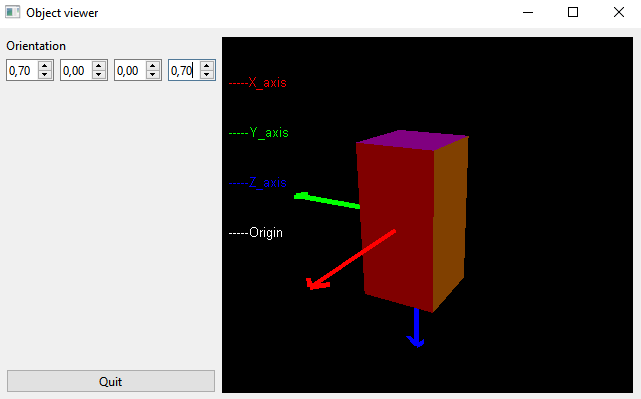This is the problem I’m facing and don’t know how to fix it.

Note that (0,0,0,0) isn’t a valid unit quaternion. The sum of the squares of the components should always be 1. The identity quaternion is (1,0,0,0).

It would have been helpful if you’d posted the view with this transformation and an identity quaternion.

However: one thing to note from the final image: the orientation of the axes no longer matches the orientation of the cube, which suggests that you aren’t using the same transformation for both.

You’ll need to clarify exactly what you’re doing with the transformations.

Okay here is my scene before changing the axes orientation: the initial one: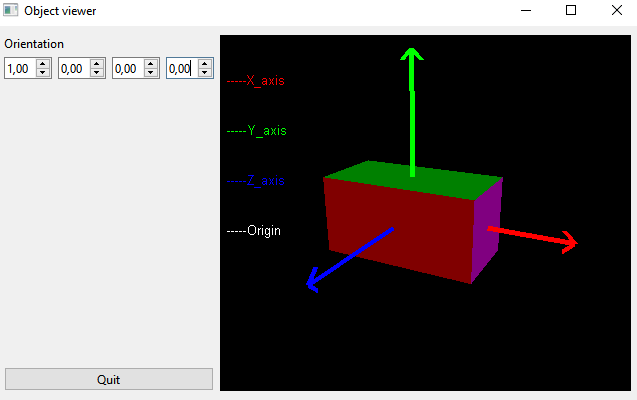Now, here is my scene after using glRotatef(-90.0f,0.0,1.0,0.0), the x and z axes’orientation changes as shown in this image below: (I haven’t applied any rotation yet)Okay I’m going to clarify exactly what I want to do with the transformation.
At first, I wanted to the object to rotate around that coordinate system(shown in the first picture) using quaternion.
I mean, when I set the values of the quaternion, in the mainwindow, as follows:
w=0.7, x=0,y=0,z=0.7, the object rotates around the z axis because these quaternion values correspond to a 90 degree rotation around the z axis.

Now, I want to change the orientation of that frame in such a way that the x and z axes can be represented as shown in the image below:So I used glRotated around the y axis to make it possible.

My main goal now is to make the object rotate around the new frame using quaternion.
When I set the quaternion values as follows: w=0.7, x=0,y=0 and z=0.7, the object is supposed to rotate around the new z axis (the blue one) with an angle of 90 degrees, however it doesn’t rotate around the new z axis but around the new x axis(the red one)which has the same orientation as the z-axis. It seems like the object keeps rotating around another frame.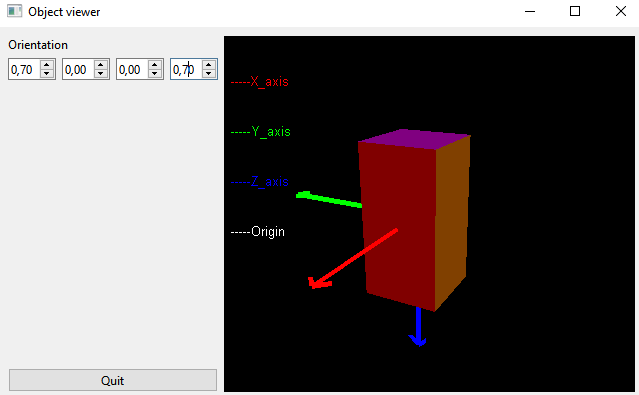I want my object to rotate around the new frame (the frame with the new orientation) which seemed confusing and hard to do.

And this is with

``````glRotatef(-90.0f,0.0,1.0,0.0);
glMultMatrixf(...);
``````

without any other matrix operations in between?

Have you checked the data which is being passed to `glMultMatrix`? It should be:

`{0,1,0,0, -1,0,0,0, 0,0,1,0, 0,0,0,1}`

(or close to it). If it isn’t, the quaternion->matrix conversion is incorrect.

For a rotation, getting the ordering wrong (row-major versus column-major) should only swap the direction of rotation, the axis should still be correct.

I used `glRotatef(-90.0f,0.0,1.0,0.0);` to rotate the frame and `glMultMatrixf` to rotate the object.
Here is exactly what I did:

``````GLWidget::GLWidget(QWidget *parent):QOpenGLWidget(parent)

{
x = 0.0; // started with the initialization of the quaternion's components
y = 0.0;
z = 0.0;
w = 1.0;

connect(&timer, SIGNAL(timeout()), this, SLOT(update()));

timer.start(16);
}
``````

then I developped a function that determines the rotation matrix from a quaternion.

Then here is my painGL function :

``````void GLWidget::paintGL()
{
glClear(GL_COLOR_BUFFER_BIT);
glClear(GL_COLOR_BUFFER_BIT | GL_DEPTH_BUFFER_BIT);
glEnable(GL_BLEND);
glBlendFunc(GL_SRC_ALPHA, GL_ONE_MINUS_SRC_ALPHA);

glMatrixMode(GL_MODELVIEW);

// camera transformation
gluLookAt(6.0, 6.0, 6.0, 3.0,3.5,0.0, 0.0,1.0,0.0);
glTranslatef(1.1, 2.1, -3.1);

glLineWidth(5.0f);
glPushMatrix();
// convert to unit quaternion (length = 1)

float qs = 1.0;
float qx = 0.0;
float qy = 0.0;
float qz = 0.0;
float ql = sqrt(w * w + x * x + y * y + z * z);
if(fabs(ql) > 0.0)
{
qs = w / ql;
qx = x / ql;
qy = y / ql;
qz = z / ql; }
float rot; // rotation matrix
getRotationFromUnitQuaternion(rot, qs, qx, qy, qz); // calculating the rotation matrix

glMultMatrixf(rot); // applying the rotation to the modelview matri

Draw_Box(); // function that draws the object
glRotatef(-90.0f,0.0,1.0,0.0); // changing the x and z axes'orientation before drawing the frame
Draw_Frame(); // a function that draws the frame

glPopMatrix();

}
``````

Also how can I check the data that’s being passed to `glMultMatrix` ? `glMultMatrixf` multiplies the modelview matrix with the rotation matrix.

So the `glRotatef` only affects the frame, not the box. If you want it to affect the coordinate system in which the quaternion rotates, it needs to come before the `glMultMatrix`.

With a debugger. Set a breakpoint on the `glMultMatrix` call and inspect `rot` when the breakpoint triggers.

1 Like

@GClements Hello, I hope you’re doing very well.

Well, that did work perfectly.

2 months ago, I drew the object and then I drew the frame which is attached to it. I did exactly as you suggested and the object rotated around the axes of the new coordinate system ( the coordinate system which I changed the orientation of its axes).

Now, here is what I did.

I started drawing the standard coordinate system, then applied transformations( I changed the orientation of its axes using rotations) . This coordinate system is a fixed one.

`````` QMatrix4x4 localMatrix;

glMatrixMode(GL_PROJECTION);

glMatrixMode(GL_MODELVIEW);
gluLookAt(2.0,2.0,0.0, 0.0,0.0,-5.0,0.0,1.0,0.0);

glTranslatef(0.0,0.0,-5.0);

glRotatef(180.0,0.0,1.0,0.0); // changing the orientation of its axes
glRotatef(-90.0,1.0,0.0,0.0); // again changing the orientation of its axes

glScalef(0.4,0.4,0.4);

DrawOrbitalFrame(); // drawing the fixed coordinate system

``````

Then I drew the textured object using shaders.

My goal was to make the object rotate around the axes of the fixed coordinate system ( after changing its axes orientation) using quaternions of course.

However when I run my program and set the quaternion values as follows:
w=0.7, x=0.0, y=0.7, z=0.0, which is equivalent to a rotation of 90° around the y-axis.

The object rotates around the y-axis of the standard coordinate system ( the default OpenGL coordinate system) and not the y-axis of the fixed coordinate system I drew and changed its axes orientation.

So I thought about applying the same transformations as I did when drawing the fixed coordinate system at first, before drawing the object:
I did this:

``````    localMatrix.rotate(180.0,0.0,1.0,0.0); // same rotation as the one applied to the coordinate system at first
localMatrix.rotate(-90.0,1.0,0.0,0.0); // same rotation as the one applied to the coordinate system at first

localMatrix.rotate(quaternion);
Then I drew the object
``````

And that made the object rotate around the new axes of the coordinate system which is the result I’m looking for.

However, I still don’t get why it worked.
If I remove the transformations before drawing the object, apply rotation using a quaternion, the object goes back to rotating around the default OpenGL coordinate system which is the one represented in the picture below: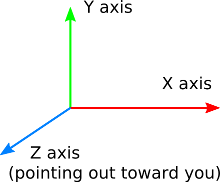This topic was automatically closed 183 days after the last reply. New replies are no longer allowed.